Cody

# Problem 42476. Model a falling body

An object is falling freely from a height of 22 meters under the force of gravity.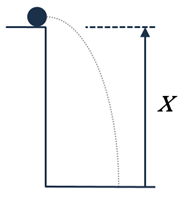Ignoring wind resistance, draw a diagram to compute the change in position, x(t), over time given the following equation of motion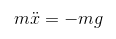Stop the simulation when the object hits the ground. The initial height and mass of the falling object are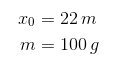and the standard acceleration due to gravity is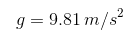### Solution Stats

47.45% Correct | 52.55% Incorrect
Last solution submitted on Mar 12, 2019

#### More from this Author14

You Can Use Simulink

#### Tags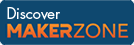MATLAB and Simulink resources for Arduino, LEGO, and Raspberry Pi